# Precalculus : Find a Point of Discontinuity

## Example Questions

← Previous 1 2 Next →

### Example Question #11 : Find A Point Of Discontinuity

Find the point of discontinuity for the following function: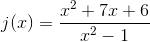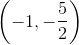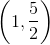There is no point of discontinuity for this function.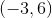Explanation:

Start by factoring the numerator and denominator of the function.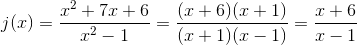A point of discontinuity occurs when a numberis both a zero of the numerator and denominator.

Since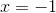is a zero for both the numerator and denominator, there is a point of discontinuity there. To find thevalue, plug ininto the final simplified equation.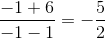is the point of discontinuity.

### Example Question #12 : Find A Point Of Discontinuity

Find the point of discontinuity for the following function: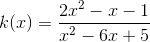There is no point of discontinuity for this function.Explanation:

Start by factoring the numerator and denominator of the function.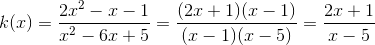A point of discontinuity occurs when a numberis both a zero of the numerator and denominator.

Sinceis a zero for both the numerator and denominator, there is a point of discontinuity there. To find thevalue, plug ininto the final simplified equation.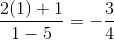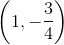is the point of discontinuity.

### Example Question #13 : Find A Point Of Discontinuity

Find the point of discontinuity for the following function: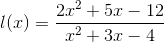There is no point of discontinuity for this function.Explanation:

Start by factoring the numerator and denominator of the function.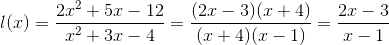A point of discontinuity occurs when a numberis both a zero of the numerator and denominator.

Since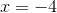is a zero for both the numerator and denominator, there is a point of discontinuity there. To find thevalue, plug ininto the final simplified equation.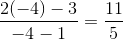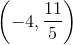is the point of discontinuity.

### Example Question #41 : Polynomial Functions

Find the point of discontinuity for the following function: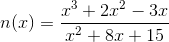There is no point of discontinuity for this function.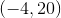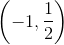Explanation:

Start by factoring the numerator and denominator of the function.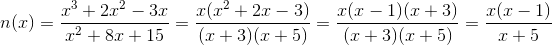A point of discontinuity occurs when a numberis both a zero of the numerator and denominator.

Since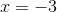is a zero for both the numerator and denominator, there is a point of discontinuity there. To find thevalue, plug ininto the final simplified equation.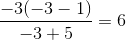is the point of discontinuity.

### Example Question #11 : Find A Point Of Discontinuity

Find the point of discontinuity for the following function: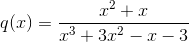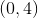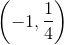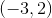There is no discontinuity for this function.Explanation:

Start by factoring the numerator and denominator of the function.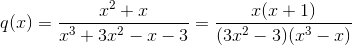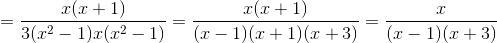A point of discontinuity occurs when a numberis both a zero of the numerator and denominator.

Sinceis a zero for both the numerator and denominator, there is a point of discontinuity there. To find thevalue, plug ininto the final simplified equation.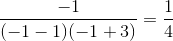is the point of discontinuity.

### Example Question #16 : Find A Point Of Discontinuity

Given the function,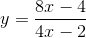, where and what is the type of discontinuity, if any?Explanation:

Before we simplify, set the denominator equal to zero to determine whereis invalid.  The value of the denominator cannot equal to zero.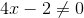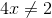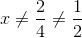The value at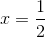is invalid in the domain.

Pull out a greatest common factor for the numerator and the denominator and simplify.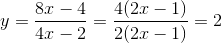Since the terms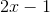can be cancelled, there will not be any vertical asymptotes.  Even though the rational function simplifies to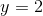, there will be instead a hole aton the graph.

The answer is: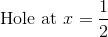### Example Question #17 : Find A Point Of Discontinuity

Find the point of discontinuity in the function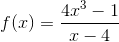.When dealing with a rational expression, the point of discontinuity occurs when the denominator would equal 0. In this case,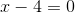so. Therefore, your point of discontinuity is.